# CBSE 10th Chemistry | Chemical Reactions and Equations -NCERT Questions with Answers

## Chemical Reactions and Equations -NCERT Questions with Answers

### Question 1. Which of the statements about the reaction below are incorrect?

#### (d) Lead oxide is getting reduced

##### (iv) all

The statements (a) and (b) are incorrect because PbO is getting reduced, not Pb, similarly C is getting oxidized not CO2.

### The above reaction is an example of a

#### (d) displacement reaction.

(d) The given reaction is an example of displacement reaction as in this reaction Al displaces, Fe from Fe2O3.

### Question 4. What is a balanced chemical equation? Why should chemical equations be balanced?

Balanced chemical equation: – A chemical equation is said to be balanced if the number of atoms of each element participating in the reaction are equal on both side of the equation.

The chemical reaction should be balanced because law of conservation of mass holds good which states that “in a chemical reaction total mass of the reactant must be equal to the total mass of the product”.

### Quiestion 5. Translate the following statements into chemical equations and then balance them.

#### (d) Potassium metal reacts with water to give potassium hydroxide and hydrogen gas.

(c) 3BaCl2(aq) + Al2 (SO4)3(s)3BaSO4(s) + 2AlCl3(aq)

### Question 8. Write the balanced chemical equation for the following and identify the type of reaction in each case.

#### (d) Magnesium(s) + Hydrochloric acid(aq)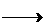Magnesium chloride(aq) + Hydrogen(g)

(a) 2KBr(aq) + Bal2(aq)2KI(aq) + BaBr2(aq)

(d) Mg(s) + 2HCl(aq)MgCl2(aq) + H2(g)

### Question 9. What does one mean by exothermic and endothermic reaction? Give examples.

Exothermic reaction: – Those reactions which occur with evolution of heat are exothermic reactions. e.g.

2NaOH(aq) + H2SO4(aq)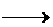Na2SO4(aq) + 2H2O(l) + Q

Enothermic reaction: – Those reactions which require heat to occur are endothermic reactions, e.g.

2Pb(NO3)2(s) + Q2PbO(s) + 4NO2(g) + O2(g)

### Question 10. Why is respiration considered an exothermic reaction? Explain.

During respiration oxidation of glucose occur which produce heat energy.

### Question 11. Why are decomposition reactions called the opposite of combination reactions? Write equations for these reactions.

During decomposition reaction a single reactant decompose to form two or more products, whereas in combination two or more reactant react to form a single product, e.g.

2H2O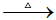2H2 + O2 (Decomposition reaction.)

### Question 12. Write one equation each for decomposition reactions where energy is supplied in the form of heat, light or electricity.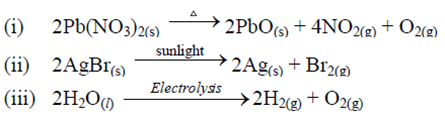### Question 13. What is the difference between displacement and double displacement reactions? Write equations for these reactions.

Displacement reaction: In this reaction an element which is more active displaces the other element which is less active from its salt solution.

e.g. Fe(s) + CuSO4(aq)FeSO4(aq) + Cu(s)

Double displacement reaction: In this reaction exchange of ions between two reactants take place, e.g.

BaCl2(aq) + Na2SO4(aq)BaSO4(s) + 2NaCl(aq)

### Question 14. In the refining of silver, the recovery of silver from silver nitrate solution involved displacement by copper metal. Write down the reaction involved.

Cu(s) + 2AgNO3(aq)Cu(NO3)2(aq) + 2Ag(s)

### Question 15. What do you mean by a precipitation reaction? Explain by giving examples.

Those reactions which produce an isoluble product or precipitate are known as precipitation reaction. e.g.AgNO3(aq) + NaCl(aq)AgCl(s) +NaNO3(aq)

### Question 16. Explain the following in terms of gain or loss of oxygen with two examples each.

#### (b) Reduction

Oxidation: Oxidation involve addition of oxygen e.g.

Reduction: Reduction involve removal of oxygen, e.g.

C + H2OCO + H2, H2O is reduced to H2.

CuO + H2Cu + H2O, CuO is reduced to Cu.

### Question 17. A shiny brown coloured element ‘X’ on heating in air becomes black in colour. Name the element ‘X’ and the black coloured compound formed.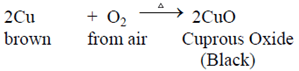### Question 18. Why do we apply paint on iron articles?

To prevent iron articles from rusting, we apply paint on it, by which iron does not come in contact with air and moisture which cause rusting.

### Question 19. Oil and fat containing food items are flushed with nitrogen. Why?

When the oil and fat containing food is surrounded by unreactive gas nitrogen, there is no oxygen to cause its oxidation and make it rancid.